# Select a row of series or dataframe by given integer index

• Last Updated : 13 Sep, 2022

The Dataframe.iloc[] is used to select a row of Series/Dataframe by a given integer index in Python

## Creating DataFrame to Select a Row by Index

Here, we are creating a Pandas Dataframe with ID, Product, Price, Color, and Discount with there some values in it.

## Python3

 `# import pandas library``import` `pandas as pd` `# Create the dataframe``df ``=` `pd.DataFrame({``'ID'``: [``'114'``, ``'345'``,``                         ``'157788'``, ``'5626'``],``                ``'Product'``: [``'shirt'``, ``'trousers'``,``                           ``'tie'``, ``'belt'``],``                ``'Price'``: [``1200``, ``1500``,``                         ``600``, ``352``],``                ``'Color'``: [``'White'``,``'Black'``,``                         ``'Red'``, ``'Brown'``],``                ``'Discount'``: [``10``, ``10``,``                            ``10``, ``10``]})` `# Show the dataframe``df`

Output: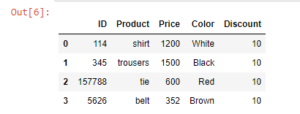## Select the first row only

Here, we are selecting the first rows using iloc, Dataframe.iloc[] method is used when the index label of a data frame is something other than numeric series.

## Python3

 `# select first row``# from the dataframe``df.iloc[``0``]`

Output: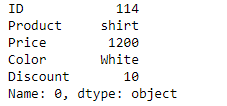## Selecting  0, 1, 2  rows.

Here, we are selecting the first second, and third rows using iloc[0:3], starting with 0 indexes and ending with (3-1=2) index.

## Python3

 `# select 0, 1, 2 rows``#from the dataframe``df.iloc[``0` `: ``3``]`

Output: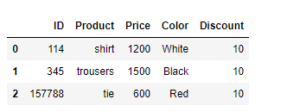## Select rows from 0 to 2 and columns from 0 to 1

Here, we are selecting the first second third rows, and the first and second columns using iloc[0:3].

## Python3

 `# selecting rows from 0 to``# 2 and columns 0 to 1``df.iloc[``0` `: ``3``, ``0` `: ``2``]`

Output: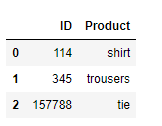## Select all rows and 2nd column

Selecting all the rows and only the second columns from the Dataframe.

## Python3

 `# selecting all rows and``# 3rd column``df.iloc[ : , ``2``]`

Output: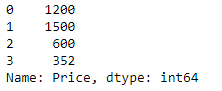## Select all rows and columns from 0 to 3.

Selecting all rows and columns.

## Python3

 `# selecting all rows and``# columns from 0 to 3``df.iloc[ : , ``0` `: ``4``]`

Output: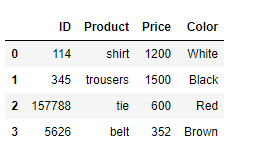My Personal Notes arrow_drop_up